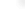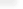## Enhanced Fourier Analysis

Mathematica 7 gives Mathematica complete coverage of symbolic Fourier analysis, adding function and sequence convolutions, Fourier series coefficients and discrete-time Fourier transforms (DTFTs), as well as adding comprehensive multivariate support—making Mathematica a unique platform for symbolic analysis of the complete range of systems where Fourier methods can be used.

Related Functions